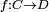# Riemann-Hurwitz formula

In algebraic geometry the Riemann-Hurwitz formula, named after Bernhard Riemann and Adolf Hurwitz, states that if C, D are smooth algebraic curves, and$f:C\to D$ is a finite map of degree d then the number of branch points of f, denoted by B, is given bya triangulated gluing diagram for the Riemann sphere, and its pullback to a torus double cover, which is ramified over the vertices of the triangulation$2 (\mbox{genus}(C)-1)=2d(\mbox{genus}(D)-1)+B. \,$

Over a field in general characteristic, this theorem is a consequence of the Riemann-Roch theorem. Over the complex numbers, the theorem can be proved by choosing a triangulation of the curve D such that all the branch points of the map are nodes of the triangulation. One then considers the pullback of the triangulation to the curve C and computes the Euler characteristics of both curves.Some content on this page may previously have appeared on Citizendium.